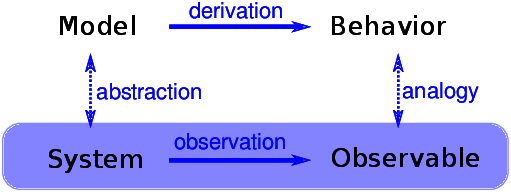# 6.9. Explanatory Models¶Figure 6.6: The logical structure of an explanatory model.

We started the discussion of networks with Milgram’s Small World Experiment, which shows that path lengths in social networks are surprisingly small; hence, “six degrees of separation”.

When we see something surprising, it is natural to ask “Why?” but sometimes it’s not clear what kind of answer we are looking for. One kind of answer is an explanatory model (see Figure 6.6). The logical structure of an explanatory model is:

1. In a system, $$S$$, we see something observable, $$O$$, that warrants explanation.

2. We construct a model, $$M$$, that is analogous to the system; that is, there is a correspondence between the elements of the model and the elements of the system.

3. By simulation or mathematical derivation, we show that the model exhibits a behavior, $$B$$, that is analogous to $$O$$.

4. We conclude that $$S$$ exhibits $$O$$ because $$S$$ is similar to $$M$$, $$M$$ exhibits $$B$$, and $$B$$ is similar to $$O$$.

5. At its core, this is an argument by analogy, which says that if two things are similar in some ways, they are likely to be similar in other ways.

Argument by analogy can be useful, and explanatory models can be satisfying, but they do not constitute a proof in the mathematical sense of the word.

Remember that all models leave out, or “abstract away”, details that we think are unimportant. For any system there are many possible models that include or ignore different features. And there might be models that exhibit different behaviors that are similar to $$O$$ in different ways. In that case, which model explains $$O$$?

The small world phenomenon is an example: the Watts-Strogatz (WS) model and the Barabási-Albert (BA) model both exhibit elements of small world behavior, but they offer different explanations:

The WS model suggests that social networks are “small” because they include both strongly-connected clusters and “weak ties” that connect clusters.

The BA model suggests that social networks are small because they include nodes with high degree that act as hubs, and that hubs grow, over time, due to preferential attachment.

As is often the case in young areas of science, the problem is not that we have no explanations, but too many.